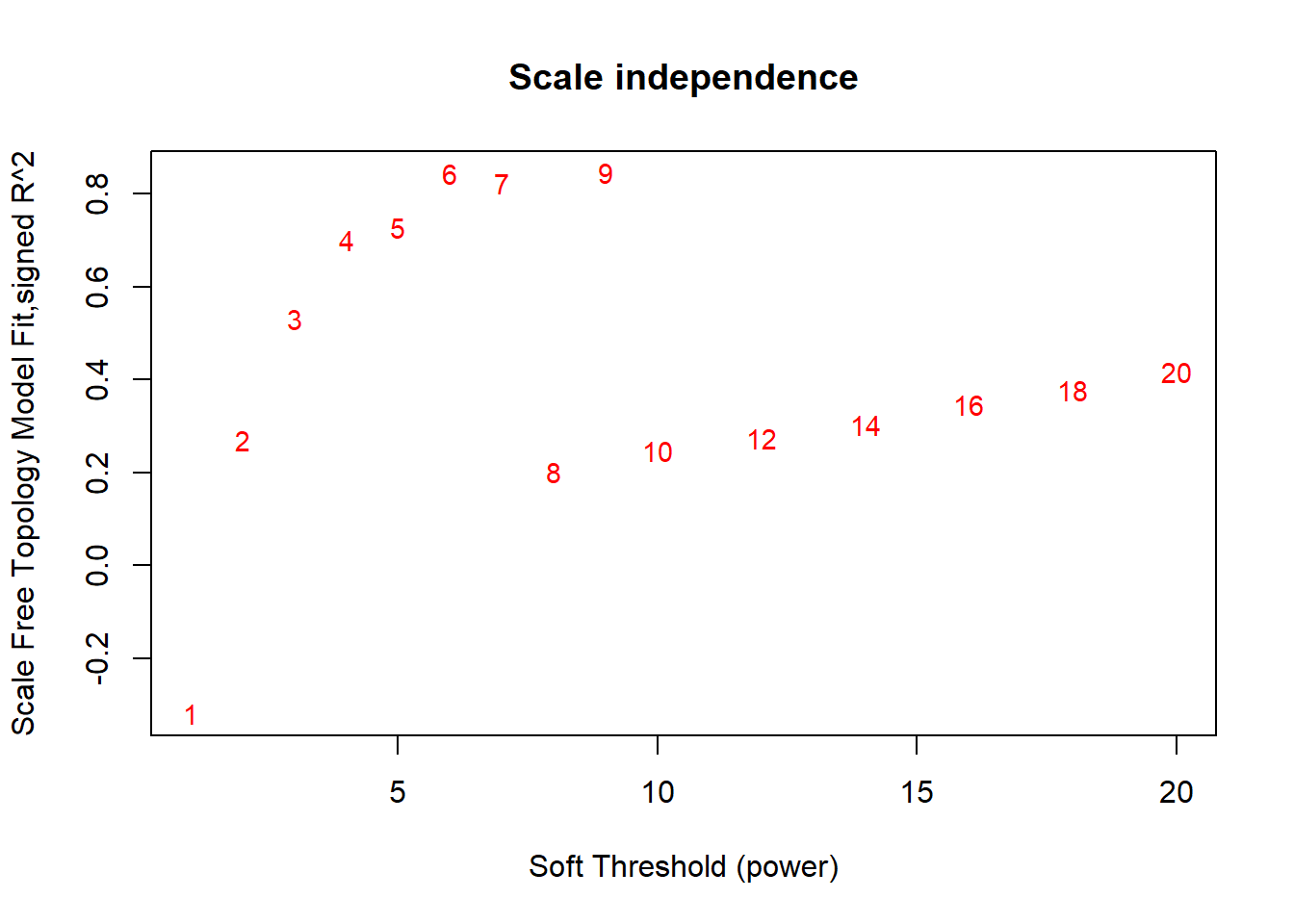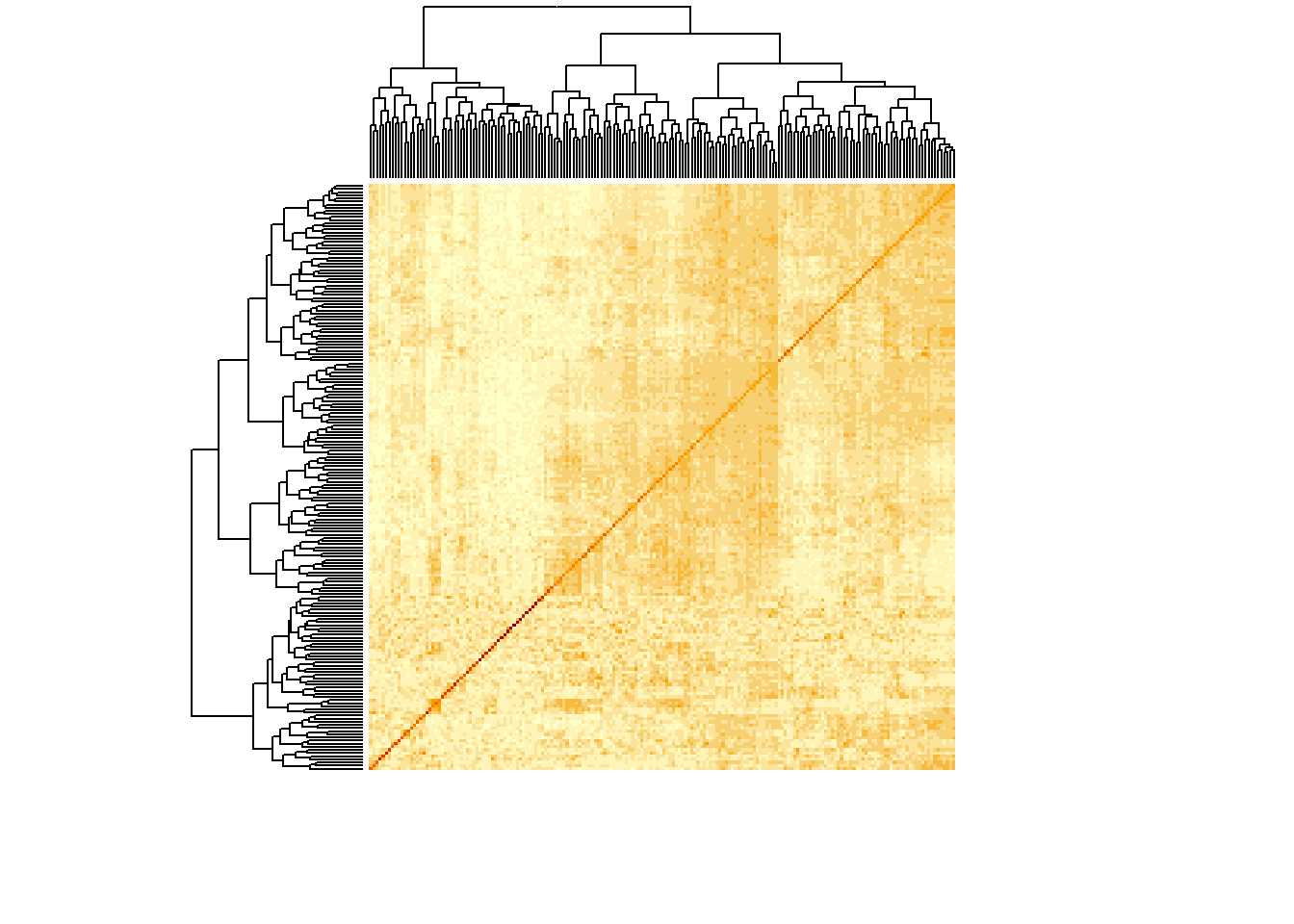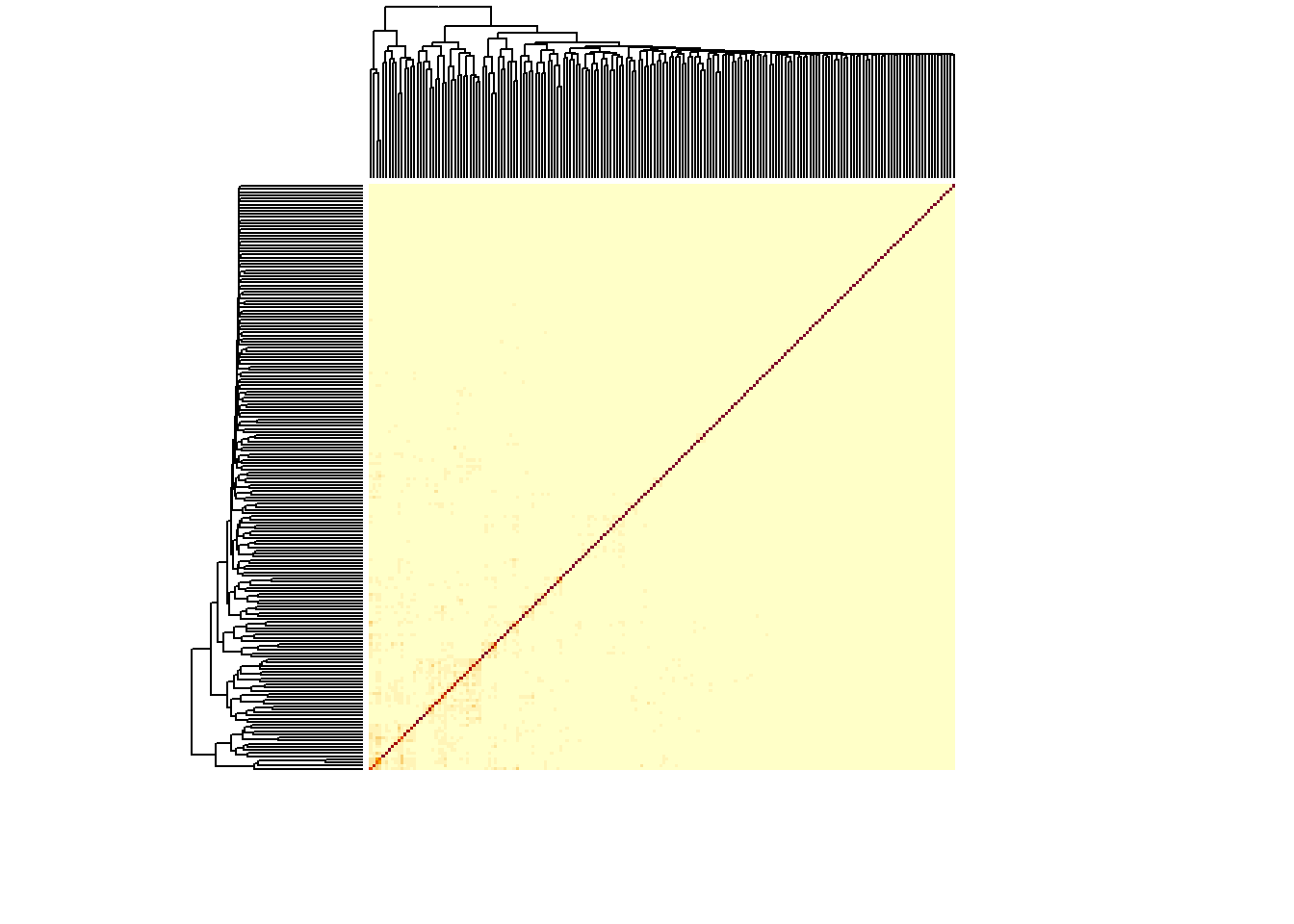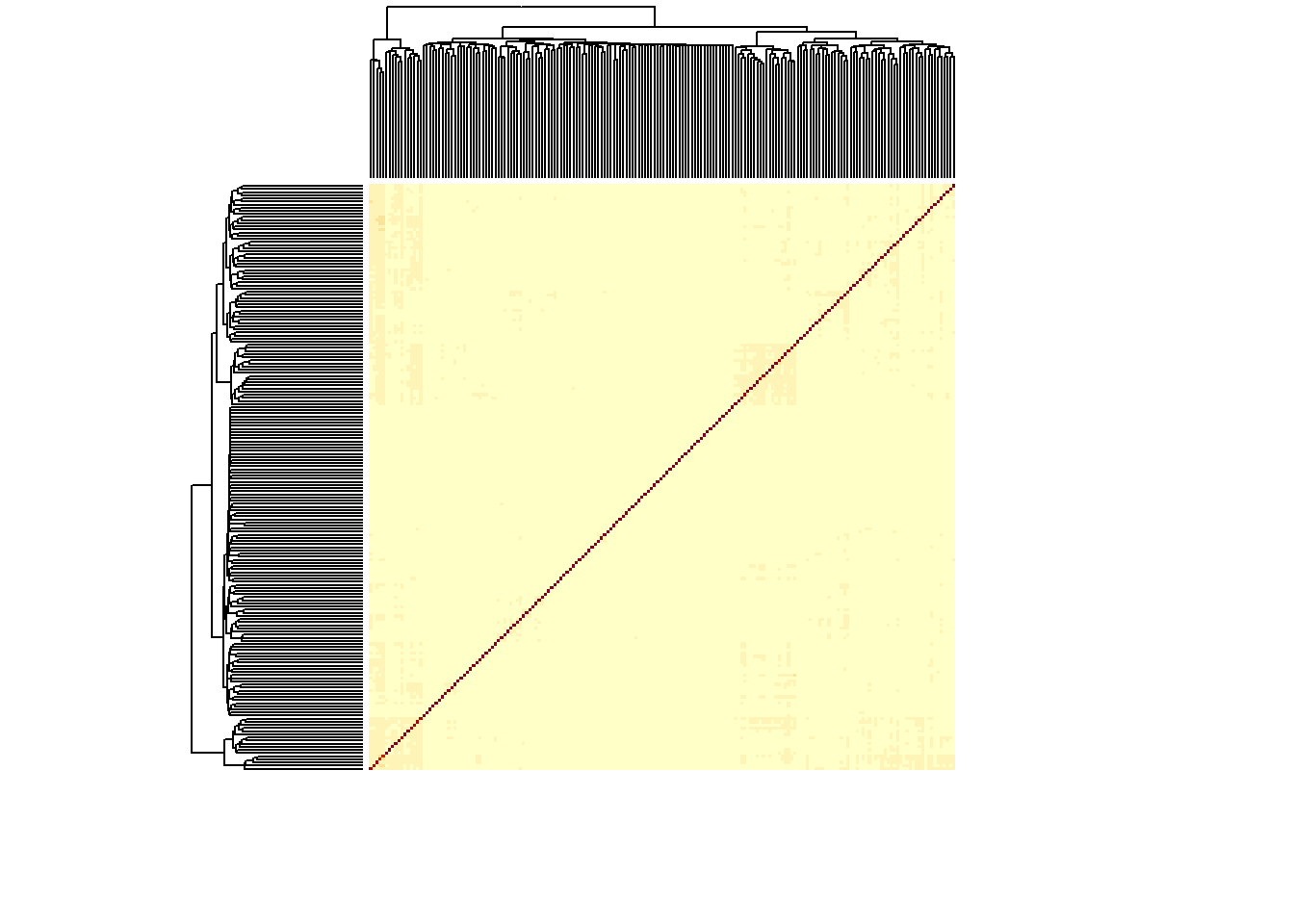## WGCNA

Although WGCNA was originally developed for gene co-expression networks, it can also be used to generate microbial co-occurrence networks. One of the major advantages of WGCNA is that it tries to find a scale-free network and identifies module memberships (Langfelder & Horvath, 2008). Therefore, this tool tends to generate networks with clear hub species, rather than a giant hairball. However, the original WGCNA tutorials do not include preprocessing steps that may be more appropriate for microbial data analysis. Therefore, this tutorial describes how to run WGCNA on a 16S rRNA dataset. Some of the code was adapted from the original WGCNA tutorials.

We are going to work on a phyloseq object that can be downloaded here. As WGCNA cannot work directly on phyloseq objects, let's extract the abundance table first. Keep in mind that WGCNA needs to have the taxa as columns.

library(WGCNA)
## Loading required package: dynamicTreeCut
## Loading required package: fastcluster
##
## Attaching package: 'fastcluster'
## The following object is masked from 'package:stats':
##
##     hclust
## 
##
## Attaching package: 'WGCNA'
## The following object is masked from 'package:stats':
##
##     cor
library(phyloseq)
library(ggplot2)
library(igraph)
##
## Attaching package: 'igraph'
## The following objects are masked from 'package:stats':
##
##     decompose, spectrum
## The following object is masked from 'package:base':
##
##     union
library(compositions)
## Welcome to compositions, a package for compositional data analysis.
## Find an intro with "? compositions"
##
## Attaching package: 'compositions'
## The following object is masked from 'package:igraph':
##
##     normalize
## The following object is masked from 'package:WGCNA':
##
##     cor
## The following objects are masked from 'package:stats':
##
##     cor, cov, dist, var
## The following objects are masked from 'package:base':
##
##     %*%, norm, scale, scale.default
### If you can't import the file:
### They are in the Workshop folder
### This file is already preprocessed!
otus <- read.table("../Workshop network analysis 2019/spiec_otus.txt")
tax <- as.matrix(read.table("../Workshop network analysis 2019/spiec_tax.txt"))
phyloseqobj.f <- phyloseq(otu_table(otus, taxa_are_rows = TRUE), tax_table(tax))

## Preprocessing the data

The abundance table is still in absolute counts - but this is not quantitative data. While SPIEC-EASI carries out a clr transform internally, WGCNA does no such thing. Therefore, we will first transform the data before analysis with the compositions package.

## Running WGCNA

Now that the data has been processed, we can construct a network. WGCNA has a convenient wrapper function that carries out all steps at once: blockwiseModules. However, let's first go through some of the steps for network construction. We can use the soft threshold function to identify what threshold will give a scale-free network topology.

powers = c(c(1:10), seq(from = 12, to=20, by=2))
sft = pickSoftThreshold(data, powerVector = powers, verbose = 5)
## pickSoftThreshold: will use block size 188.
##  pickSoftThreshold: calculating connectivity for given powers...
##    ..working on genes 1 through 188 of 188
##    Power SFT.R.sq  slope truncated.R.sq mean.k. median.k. max.k.
## 1      1    0.318  0.649         0.6640 55.6000  56.20000 88.200
## 2      2    0.270 -0.362         0.6750 22.9000  22.00000 49.100
## 3      3    0.531 -0.690         0.8210 11.0000   9.72000 30.300
## 4      4    0.700 -0.907         0.8520  5.8400   4.74000 19.700
## 5      5    0.728 -1.090         0.8760  3.3000   2.41000 13.300
## 6      6    0.844 -1.210         0.9250  1.9600   1.25000  9.320
## 7      7    0.822 -1.280         0.8610  1.2100   0.68500  6.690
## 8      8    0.201 -2.510         0.0111  0.7770   0.38200  4.930
## 9      9    0.845 -1.390         0.8580  0.5120   0.22600  3.710
## 10    10    0.247 -3.380         0.0927  0.3460   0.13100  2.860
## 11    12    0.275 -2.630         0.1760  0.1680   0.05010  1.820
## 12    14    0.304 -3.390         0.2310  0.0882   0.02110  1.250
## 13    16    0.346 -3.910         0.2500  0.0495   0.00847  0.919
## 14    18    0.378 -4.480         0.2350  0.0295   0.00359  0.709
## 15    20    0.416 -4.460         0.2510  0.0187   0.00154  0.569
plot(sft$fitIndices[,1], -sign(sft$fitIndices[,3])*sft$fitIndices[,2], xlab="Soft Threshold (power)",ylab="Scale Free Topology Model Fit,signed R^2",type="n", main = paste("Scale independence")) text(sft$fitIndices[,1], -sign(sft$fitIndices[,3])*sft$fitIndices[,2], labels=powers,cex=0.9,col="red")Normally, we would use a cutoff based on the R squared; e.g. 0.9. However, there is no soft threshold in this range that results in such a value. Therefore, we could go with 6 as a setting for the soft threshold, as the R squared does not improve significantly with a more stringent threshold. This setting is necessary to compute the adjacency matrix and adjust it to become scale-free. Let's see what the difference between 1 and 6 is.

adjacency = adjacency(data, power=1, type="unsigned")
heatmap(adjacency, labRow=FALSE, labCol=FALSE)adjacency = adjacency(data, power=6, type="unsigned")
heatmap(adjacency, labRow=FALSE, labCol=FALSE)Clearly, the thresholding function is helping to remove a large portion of predicted interactions. However, WGCNA does take into account node neighbourhoods by adjusting the matrix. It does so by calculating the topological overlap between nodes: the number of neighbours they share. The adjacency matrix needs to have values between 0 and 1; as some values are outside this range due to rounding errors, we will remove them first. The topological overlap matrix is then used to determine modules, as WGCNA carries out clustering on the TOM. Modules with highly similar eigengenes are removed.

adjacency[adjacency < 0] = 0
TOM = TOMsimilarity(adjacency)
## ..connectivity..
## ..matrix multiplication (system BLAS)..
## ..normalization..
## ..done.
heatmap(TOM, labRow=FALSE, labCol=FALSE)Let's explore the effect some of the WGCNA settings have on our dataset by running the 1-step network construction and module detection function. We'll use the TOM to construct a network and then overlay cluster assignments to see how the signed vs the unsigned TOM are different. First, we run network inference & construction on the unsigned network type.

adjacency = adjacency(data, power=6, type="unsigned")
network <- simplify(network)  # removes self-loops
results <- blockwiseModules(data, power=6, TOMType="unsigned", networkType="unsigned")
V(network)$color <- results$colors
par(mar=c(0,0,0,0))
# remove unconnected nodes
network <- delete.vertices(network, degree(network)==0)
plot(network, layout=layout.fruchterman.reingold(network), edge.arrow.size = 0.2)

Next, we do the same but set the network type and TOM type to signed.

adjacency = adjacency(data, power=6, type="signed")
V(network)$color <- results$colors
plot(network, layout=layout.fruchterman.reingold(network), edge.arrow.size = 0.2)